﻿ ehpp.html

# 2. Elliptic, Hyperbolic, Parabolic and Planar Points of a Surface

 T is elliptic if G(T) > 0 (equivalently K1 and K2 have the same sign); T is hyperbolic if G(T) < 0 (equivalently K1 and K2 have opposite signs); T is parabolic if G(T) = 0 (equivalently exactly one of K1 and K2 is zero); T is planar if G(T) = 0 (equivalently K1=K2=Kt=0).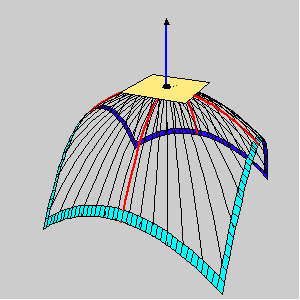an elliptic point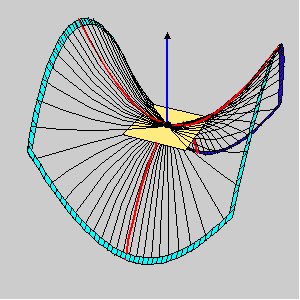a hyperbolic point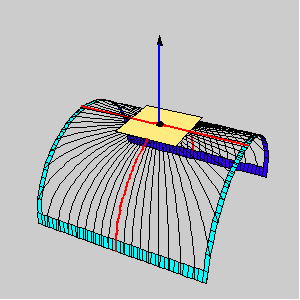a parabolic point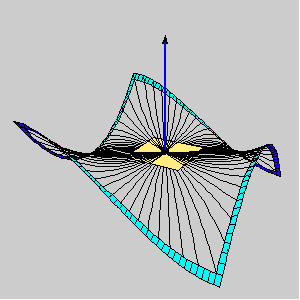a planar point

EXAMPLE: Ring-torus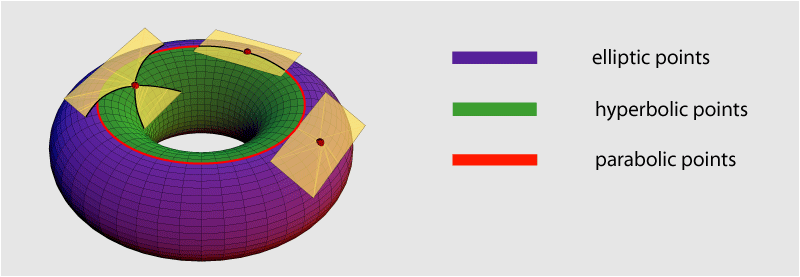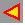back